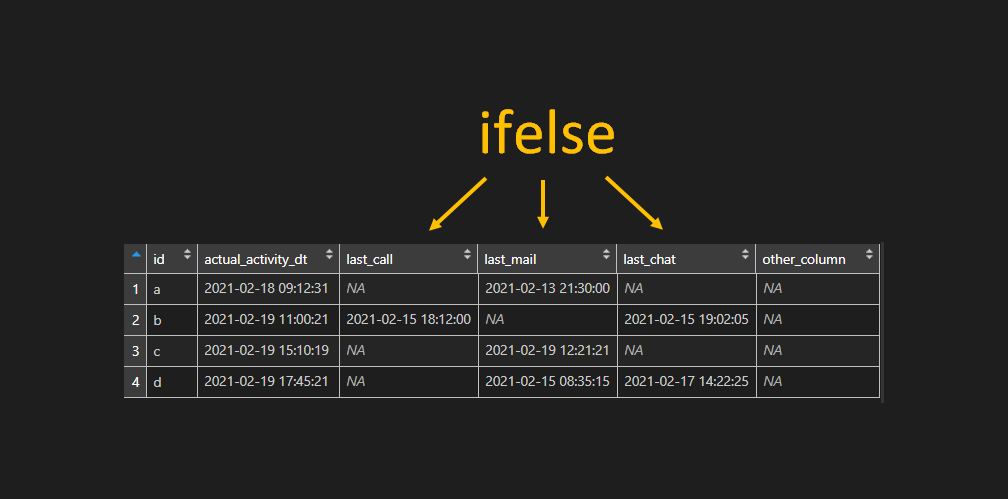# Use ifelse across a range of R data frame columns

Here is how to apply the ifelse function across a range of multiple R data frame columns. Sometimes it is necessary to do calculations by a condition and it could be time-consuming to do that for each of multiple columns. Or even worse. Maybe the necessary columns are changing position over time and you have to select necessary ones automatically.

Here is my data frame.

```df <- structure(
list(
id = structure(1:4, .Label = c("a", "b", "c", "d"), class = "factor"),
actual_activity_dt = structure(
c(1613632351, 1613725221, 1613740219, 1613749521),
class = c("POSIXct", "POSIXt"), tzone = ""
),
last_call = structure(
c(NA, 1613405520, NA, NA),
class = c("POSIXct", "POSIXt"), tzone = ""
),
last_mail = structure(
c(1613244600, NA, 1613730081, 1613370915),
class = c("POSIXct", "POSIXt"), tzone = ""
),
last_chat = structure(
c(NA, 1613408525, NA, 1613564545),
class = c("POSIXct", "POSIXt"), tzone = ""
),
other_column = c(NA, NA, NA, NA)
),
row.names = c(NA, -4L),
class = c("tbl_df", "tbl", "data.frame")
)

df
## A tibble: 4 x 6
#  id    actual_activity_dt  last_call           last_mail           last_chat           other_column
#
#1 a     2021-02-18 09:12:31 NA                  2021-02-13 21:30:00 NA                  NA
#2 b     2021-02-19 11:00:21 2021-02-15 18:12:00 NA                  2021-02-15 19:02:05 NA
#3 c     2021-02-19 15:10:19 NA                  2021-02-19 12:21:21 NA                  NA
#4 d     2021-02-19 17:45:21 NA                  2021-02-15 08:35:15 2021-02-17 14:22:25 NA```

I would like to calculate the time difference in days by subtracting each of the column values that start with the prefix “last_” from the datetime in the second column and overwrite them with that results.

### Select R data frame columns by part of the name

This is needed to automatically select columns if something is changing over ar time. It might be reasonable if you want to automate the R script. If there is a part that common that it is simple to do that with the grepl.

```grepl('last_', names(df))
# FALSE FALSE  TRUE  TRUE  TRUE FALSE```

In this case, there is possible to use the startsWith function that gives the same result.

```startsWith(names(x), 'last_')
# FALSE FALSE  TRUE  TRUE  TRUE FALSE```

## ifelse across a range of R data frame columns

If you have a location of necessary columns that should be transformed by using function ifelse, then it could be done with the function lapply.

```loc <- grepl('last_', names(df))

df[loc] <-
lapply(df[loc], function(x) {
ifelse(is.na(x),
NA,
difftime(df\$actual_activity_dt, x, units = 'days'))
})

df
## A tibble: 4 x 6
#  id    actual_activity_dt  last_call last_mail last_chat other_column
#
#1 a     2021-02-18 09:12:31     NA        4.49      NA    NA
#2 b     2021-02-19 11:00:21      3.70    NA          3.67 NA
#3 c     2021-02-19 15:10:19     NA        0.117     NA    NA
#4 d     2021-02-19 17:45:21     NA        4.38       2.14 NA```

### ifelse across a range of column with dplyr

If you like to work with dplyr then there is a function across that makes it easy to apply transformations to multiple columns.

For example, if you want to do the same transformation for the range of columns with as.Posixct it could be done like this.

`df <- df %>% mutate(across(2:5, as.POSIXct))`

With the original data frame the same example with ifelse looks like this.

```x %>% mutate(across(starts_with("last_"), ~ifelse(is.na(.x), NA, difftime(actual_activity_dt, .x, units = 'days'))))

## A tibble: 4 x 6
#  id    actual_activity_dt  last_call last_mail last_chat other_column
#
#1 a     2021-02-18 09:12:31     NA        4.49      NA    NA
#2 b     2021-02-19 11:00:21      3.70    NA          3.67 NA
#3 c     2021-02-19 15:10:19     NA        0.117     NA    NA
#4 d     2021-02-19 17:45:21     NA        4.38       2.14 NA```

Here are a few other examples with function across that might be helpful.

If you like to use functions from R apply family then here is a bunch of examples from DataCornering.

Variance or other calculation across columns in R

Combine apply and match in R, return the name of the column

How to combine files with R and add filename column

How to combine numbers and find sums with R

Check if a column has a missing values (NA) in R

Categories R